# Hierarchical Clustering: Foundational Concepts and Example of Agglomerative Clustering

## Recent Posts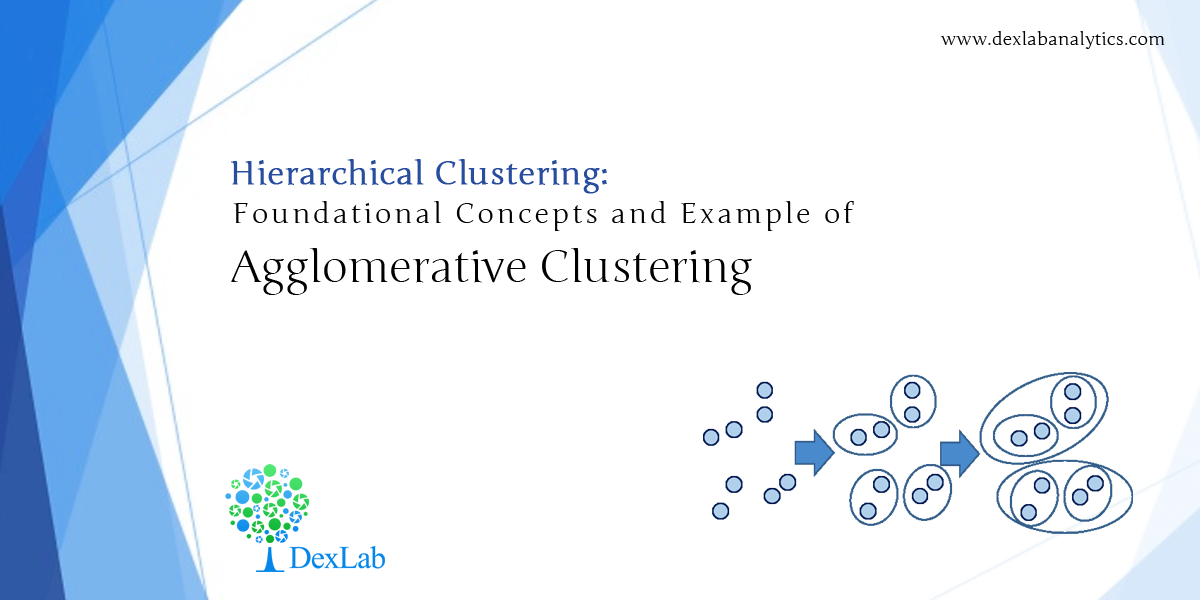Clustering is the process of organizing objects into groups called clusters. The members of a cluster are ‘’similar’’ between them and ‘’dissimilar’’ to members of other groups.

In the previous blog, we have discussed basic concepts of clustering and given an overview of the various methods of clustering. In this blog, we will take up Hierarchical Clustering in greater details.

#### Hierarchical Clustering:

Hierarchical Clustering is a method of cluster analysis that develops a hierarchy (ladder) of clusters. The two main techniques used for hierarchical clustering are Agglomerative and Divisive.

#### Agglomerative Clustering:

In the beginning of the analysis, each data point is treated as a singleton cluster. Then, clusters are combined until all points have been merged into a single remaining cluster. This method of clustering wherein a ‘’bottom up’’ approach is followed and clusters are merged as one moves up the hierarchy is called Agglomerative clustering.

The clustering is done with the help of linkage types. A particular linkage type is used to get the distance between points and then assign it to various clusters. There are three linkage types used in Hierarchical clustering- single linkage, complete linkage and average linkage.

Single linkage hierarchical clustering: In this linkage type, two clusters whose two closest members have the shortest distance (or two clusters with the smallest minimum pairwise distance) are merged in each step.

Complete linkage hierarchical clustering: In this type, two clusters whose merger has the smallest diameter (two clusters having the smallest maximum pairwise distance) are merged in each step.

Average linkage hierarchical clustering: In this type, two clusters whose merger has the smallest average distance between data points (or two clusters with the smallest average pairwise distance), are merged in each step.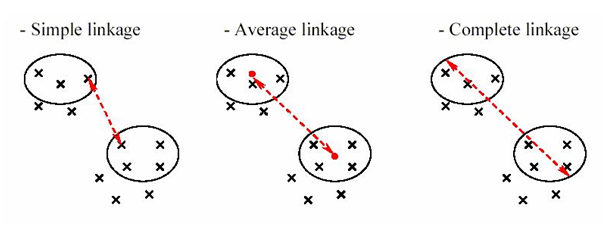Single linkage looks at the minimum distance between points, complete linkage looks at the maximum distance between points while average linkage looks at the average distance between points.

Now, let’s look at an example of Agglomerative clustering.

The first step in clustering is computing the distance between every pair of data points that we want to cluster. So, we form a distance matrix. It should be noted that a distance matrix is symmetrical (distance between x and y is the same as the distance between y and x) and has zeros in its diagonal (every point is at a distance zero from itself). The table below shows a distance matrix- only lower triangle is shown an as the upper one can be filled with reflection.Next, we begin clustering. The smallest distance is between 3 and 5 and they get merged first into the cluster ‘35’.

After this, we replace the entries 3 and 5 by ‘35’ and form a new distance matrix. Here, we are employing complete linkage clustering. The distance between ‘35’ and a data point is the maximum of the distance between the specific data point and 3 or the specific data point and 5. This is followed for every data point. For example, D(1,3)=3 and D(1,5) =11, so as per complete linkage clustering rules we take D(1,’35’)=11. The new distance matrix is shown below.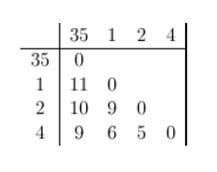Again, the items with the smallest distance get clustered. This will be 2 and 4. Following this process for 6 steps, everything gets clustered. This has been summarized in the diagram below. In this plot, y axis represents the distance between data points at the time of clustering and this is known as cluster height.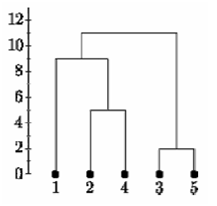If single linkage clustering was used for the same distance matrix, then we would get a single linkage dendogram as shown below. Here, we start with cluster ‘35’. But the distance between ‘35’ and each data point is the minimum of D(x,3) and D(x,5). Therefore, D(1,’35’)=3.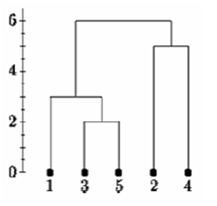Agglomerative hierarchical clustering finds many applications in marketing. It is used to group customers together on the basis of product preference and liking. It effectively determines variations in consumer preferences and helps improving marketing strategies.

In the next blog, we will explain Divisive clustering and other important methods of clustering, like Ward’s Method. So, stay tuned and follow Dexlab Analytics. We are a leading big data Hadoop training institute in Gurgaon. Enroll for our expert-guided certification courses on big data Hadoop and avail flat 10% discount!#### Interested in a career in Data Analyst?### Call us to know more### Gurgaon+91 931 572 5902